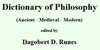### TetractysThe tetractys (τετρακτύς), or tetrad, is a triangular figure consisting of ten points arranged in four rows: one, two, three, and four points in each row, which is the geometrical representation of the fourth triangular number. As a mystical symbol, it was very important to the secret worship of the Pythagoreans. ==Pythagorean symbol== A ...
Found on http://en.wikipedia.org/wiki/Tetractys

### TetractysLiterally the Greek term signifies, an aggregate of four, specifically it was applied to the Pythagorean perfect number, ten, which is the sum of one, two, three, and four. -- J.J.R.
Found on http://www.ditext.com/runes/t.html
No exact match found